Email us to get an instant 20% discount on highly effective K-12 Math & English kwizNET Programs!

#### Online Quiz (WorksheetABCD)

Questions Per Quiz = 2 4 6 8 10

### MEAP Preparation - Grade 4 Mathematics1.41 Types of Fractions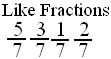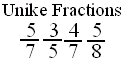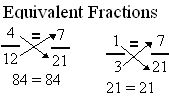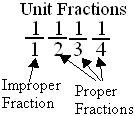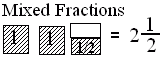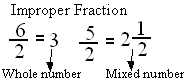Directions: Answer the following questions. Also write at least 5 examples of each type of fractions.
 Q 1: Fractions with same denominators are calledlike fractionsunlike fractions Q 2: An improper fraction is sometimes a mixed number or sometimes a whole number.TrueFalse Q 3: A fraction less than 1 is called a proper fraction. A fraction equal to one and more than 1 is called _________.improper fractionproper fractionmixed fraction Q 4: If the numerator is less than the denominator in a fraction, the fraction is called a _______.proper fractionimproper fractionmixed fraction Q 5: A number with a whole number and a proper fraction is amixed numberproper number Q 6: Fraction with numerator 1 is called a unit fraction. Are all unit fractions proper?NoYes Q 7: When a products obtained by cross multiplication give the same number, then the fractions are ____(or equal).mixedequivalentproper Q 8: If the numerator is more than the denominator in a fraction, the fraction is called a _________.improper fractionmixed fractionproper fraction Question 9: This question is available to subscribers only! Question 10: This question is available to subscribers only!